# Cycloid

(diff) ← Older revision | Latest revision (diff) | Newer revision → (diff)

The plane transcendental curve that is the trajectory of a point of a circle rolling along a straight line (Fig. a).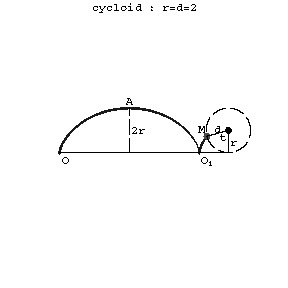Figure: c027540a

The parametric equations are: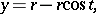whereis the radius of the circle andthe angle of rotation of the circle. In Cartesian coordinates the equation is: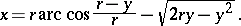A cycloid is a periodic curve: the period (basis) is. The points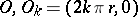,are cusps. The pointsandare the so-called vertices. The area is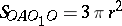, the radius of curvature is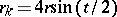.

If the curve is described by a point lying outside (inside) a circle rolling along a line, then it is called an extended, (or elongated, Fig. b), a contracted, (or shortened, Fig. c) cycloid or sometimes a trochoid.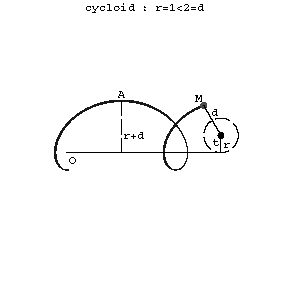Figure: c027540bFigure: c027540c

The parametric equations are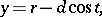whereis the distance of the pointfrom the centre of the rolling circle.

The cycloid is a tautochronic (or isochronic) curve, that is, a curve for which the time of descent of a material point along this curve from a certain height under the action of gravity does not depend on the original position of the point on the curve.